You are using an outdated browser. Please upgrade your browser to improve your experience.mathflair is a powerful learning aid to help students master rationalization of radicals, rational fractions, radical simplification, quadratic expressions and equations, simultaneous equations and much more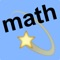mathflair school math

by Walter Kissach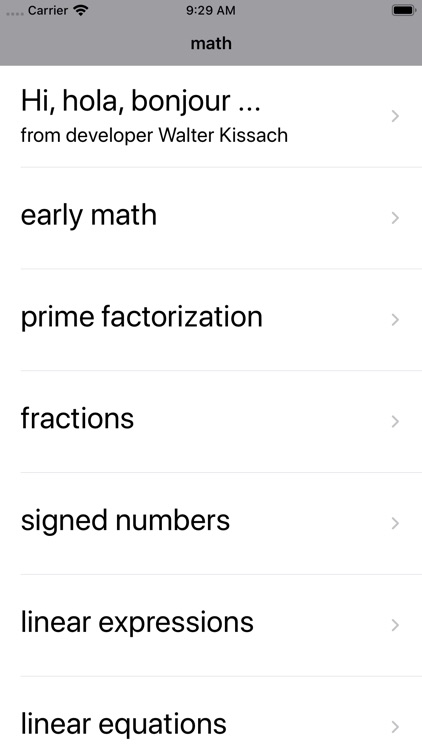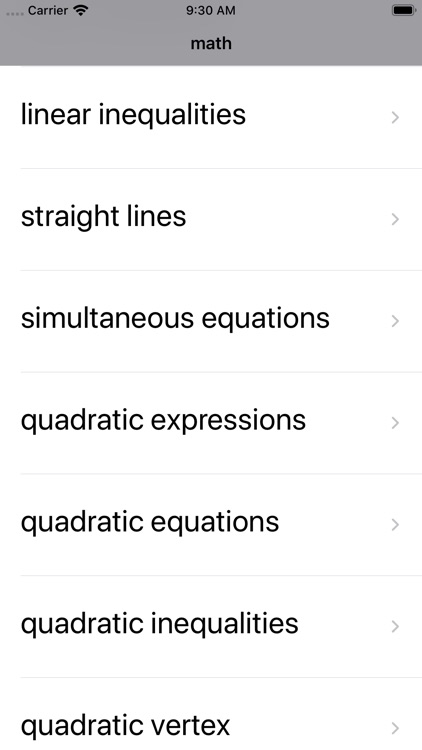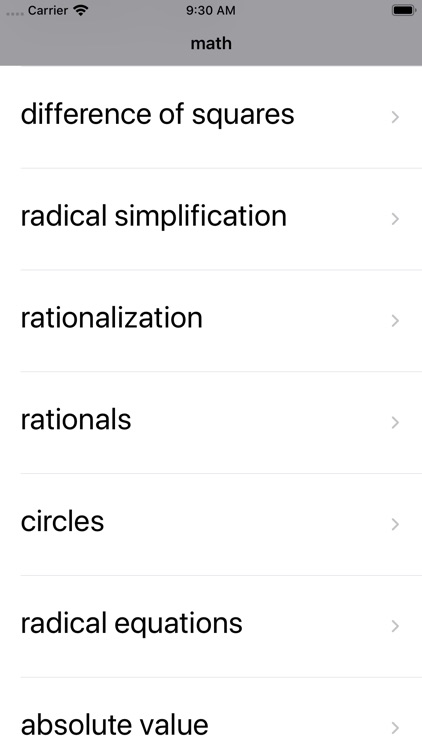What is it about?

mathflair is a powerful learning aid to help students master rationalization of radicals, rational fractions, radical simplification, quadratic expressions and equations, simultaneous equations and much more.App Details

Version
6.6
Rating
NA
Size
13Mb
Genre
Education Reference
Last updated
October 3, 2019
Release date
March 27, 2012

App Screenshots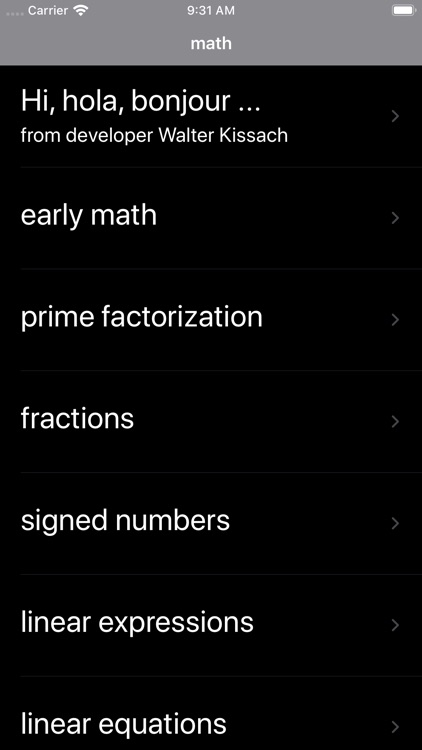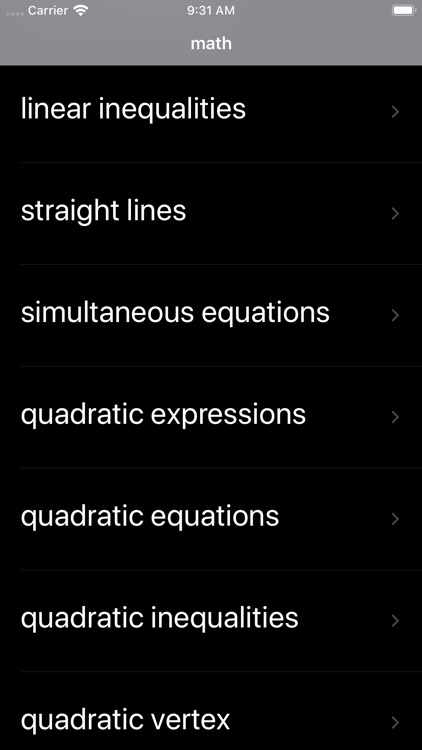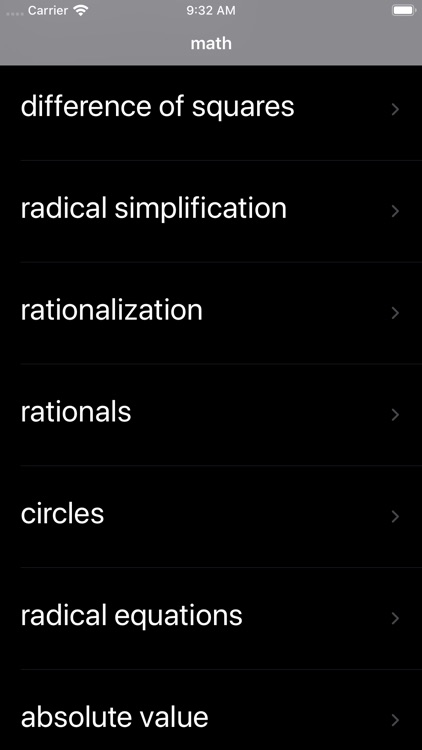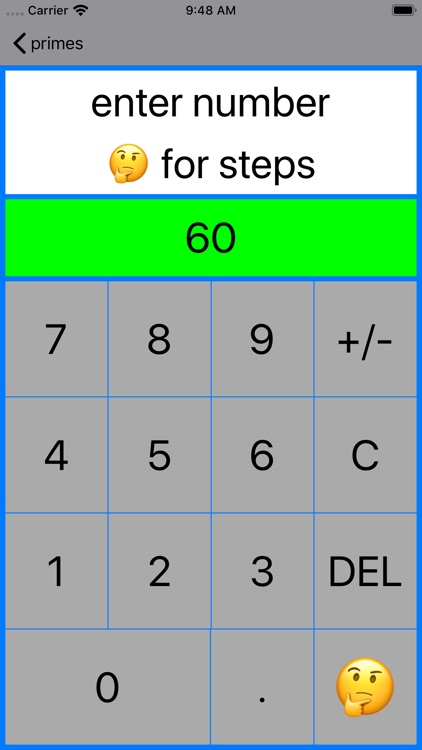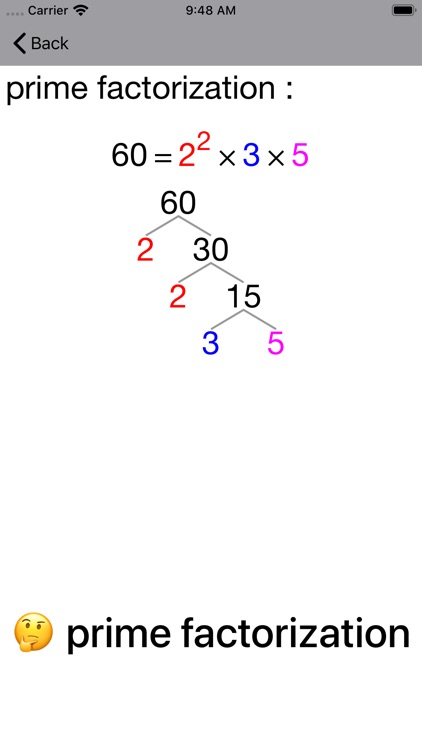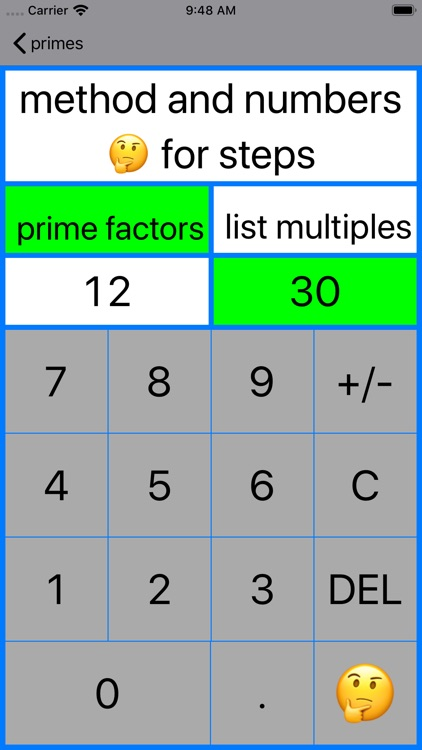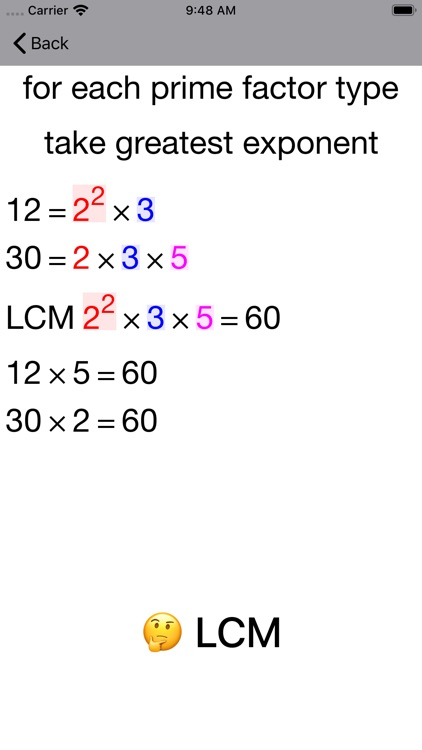App Store Description

mathflair is a powerful learning aid to help students master rationalization of radicals, rational fractions, radical simplification, quadratic expressions and equations, simultaneous equations and much more.

Interactive Coaching Calculators and Guides combine to speed the learning process.

Modern NUMBER pencil and paper methods :

- Addition with Partial Sum and Column
- Subtraction with Trade First, Count Down and Partial Difference
- Multiplication with Partial Product
- Division with Partial Quotient
- Standard algorithms

Modern FRACTION pencil and paper methods :

- Addition and subtraction in stacked or line format
- Multiplication
- Division

PRIMES

- Prime factorization by factor tree

- LCM by prime factorization
- LCM by listing multiples

- GCD by prime factorization
- GCD (GCF) by listing divisors

SIGNED NUMBERS

- addition, subtraction of two signed numbers
- multiplication, division of two signed numbers

SOLVING LINEAR EQUATIONS

- one-step
x − 2 = 3, 5 + x = 4, 3x = -12, x/7 = 2

- two-step
3x + 8 = 20, x/9 + 2 = 5

- multi-step
4 + 9x = 2x + 18

SOLVING SIMULTANEOUS LINEAR EQUATIONS

- elimination method
- substitution method

SOLVING LINEAR INEQUALITIES

- one-step
x − 2 ≤ 3, 5 + x ≥ 4, 3x ≤ -12, x/7 ≥ 2

- two-step
3x + 8 ≥ 20, x/9 + 2 ≤ 5

- multi-step
4 + 9x ≥ 2x + 18

- by Difference of Squares
- by Guess and Check method
- by Grid or Box method
- by Grouping or AC method

- by factorization
- by quadratic formula
- by completing the square

- by sketching associated graph

FINDING VERTEX OF QUADRATIC GRAPH

- by completing the square

FINDING CIRCLE CENTER AND RADIUS

- by expressing equation in centre-radius form

SIMPLIFYING SIMPLE RATIONAL FRACTIONS

- by factorization

SIMPLIFYING SQUARE ROOTS

- by perfect squares, factor trees and prime factorization

SIMPLIFYING HIGHER ROOTS

- up to sixth roots by prime factorization

RATIONALIZING RADICALS such as :

- 2/√50, 6/(8 + √20), 4/(2√5 + 3√45)

SOLVING ABSOLUTE VALUE EQUATIONS AND INEQUALITIES

- |3x − 2| = 6, |2x − 3| ≥ 5